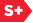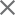Proceedings Paper

Lower bounds for finite wavelet and Gabor systems
Author(s): Ole Christensen; Alexander M. Lindner
Format Member Price Non-Member Price
PDF \$17.00 \$21.00

Paper Abstract

For a function ΦεL2(R) and parameters a>1, b>0, the corresponding wavelet family is the set of functions (function in paper). We show that for a dense set of functions (function in paper), every finite subfamily of the functions (function in paper) will be linearly independent. Under certain conditions, the result is also true for finite subsets (function in paper) of the irregular wavelet system (formula in paper), where (formula in paper). We estimate the corresponding lower Riesz bound, i.e., we find a positive number A such that (formula in paper) for all finite sequences (function in paper). We discuss cases from wavelet theory where it is crucial to have such estimates. We consider the same question for a Gabor family (formula in paper). A conjecture by Heil, Ramanathan and Topiwala states that when (formula in paper) and (formula in paper) consists of distinct points, then (formula in paper) will automatically be linearly independent. It is known that the conjecture holds in some special cases, e.g., under the assumption that g has support in a half line. We estimate the lower Riesz bound in that case.

Paper Details

Date Published: 4 December 2000
PDF: 5 pages
Proc. SPIE 4119, Wavelet Applications in Signal and Image Processing VIII, (4 December 2000); doi: 10.1117/12.408628
Show Author Affiliations
Ole Christensen, Technical Univ. of Denmark (Denmark)
Alexander M. Lindner, Univ. of Erlangen-Nuremberg (Germany)

Published in SPIE Proceedings Vol. 4119:
Wavelet Applications in Signal and Image Processing VIII
Akram Aldroubi; Andrew F. Laine; Michael A. Unser, Editor(s)